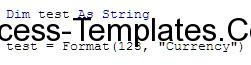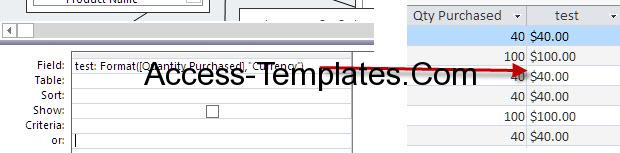# Microsoft Access Format Number Function

5 / 5 ( 1votes )

Ms Access Format Number in this tutorial will discuss the format number that can be used for fixing the number, set and determine the value type. The syntax for Format function is:

Format ( expression, [ format ] )

Expression: the (numeric) value that going to be changed.

[format]: what the value will be turned into. Despite it is the main function, it is actually optional parameter. It has several values:

 Format Explanation General Number Displays a number without thousand separators. It is the default value if the [format] parameter is omitted. Currency Displays thousand separators as well as two decimal places. Fixed Displays at least one digit to the left of the decimal place and two digits to the right of the decimal place. Standard Displays the thousand separators, at least one digit to the left of the decimal place, and two digits to the right of the decimal place. Percent Displays a percent value – that is, a number multiplied by 100 with a percent sign. Displays two digits to the right of the decimal place. Scientific Scientific notation. Yes/No Displays No if the number is 0. Displays Yes if the number is not 0. True/False Displays False if the number is 0. Displays True if the number is not 0. On/Off Displays Off if the number is 0. Displays On is the number is not 0.
Read Also:  Ms Access Query Examples on Expression Syntax Functions

Note: these are the provided format from Access. There are still other [format]’s value and you can even define your own.

Example (based on format list):

1. Format (\$1,200.00, “General Number”)

Result: 1200

1. Format (1,200, “Currency”)

Result: \$1,200.00

1. Format (1200, “Fixed”)

Result: 1200.00

1. Format (1200, “Standard”)

Result: 1,200.00

1. Format (.5, “Percent”)

Result: 50%

1. Format (40, “Scientific”)

Result: 4.00E+01

1. Format (40, ” Yes/No”)

Result: Yes

1. Format (1, “True/False”)

Result: True

1. Format (0, “On/Off”)

Result: Off

Example in VBA:Ms Access Format Number-1

There is not much difference in the VBA code, except you need to add the syntax in the first line as the argument for the return code, mainly for Data Type.

Example in query:Tutorial 2Ms Access Format Number-2. Ms Access Format Number-2

In the sample query above, we a testing field which convert the quantity in general format into currency.

#### Related Access Database

• Access format number as textTop